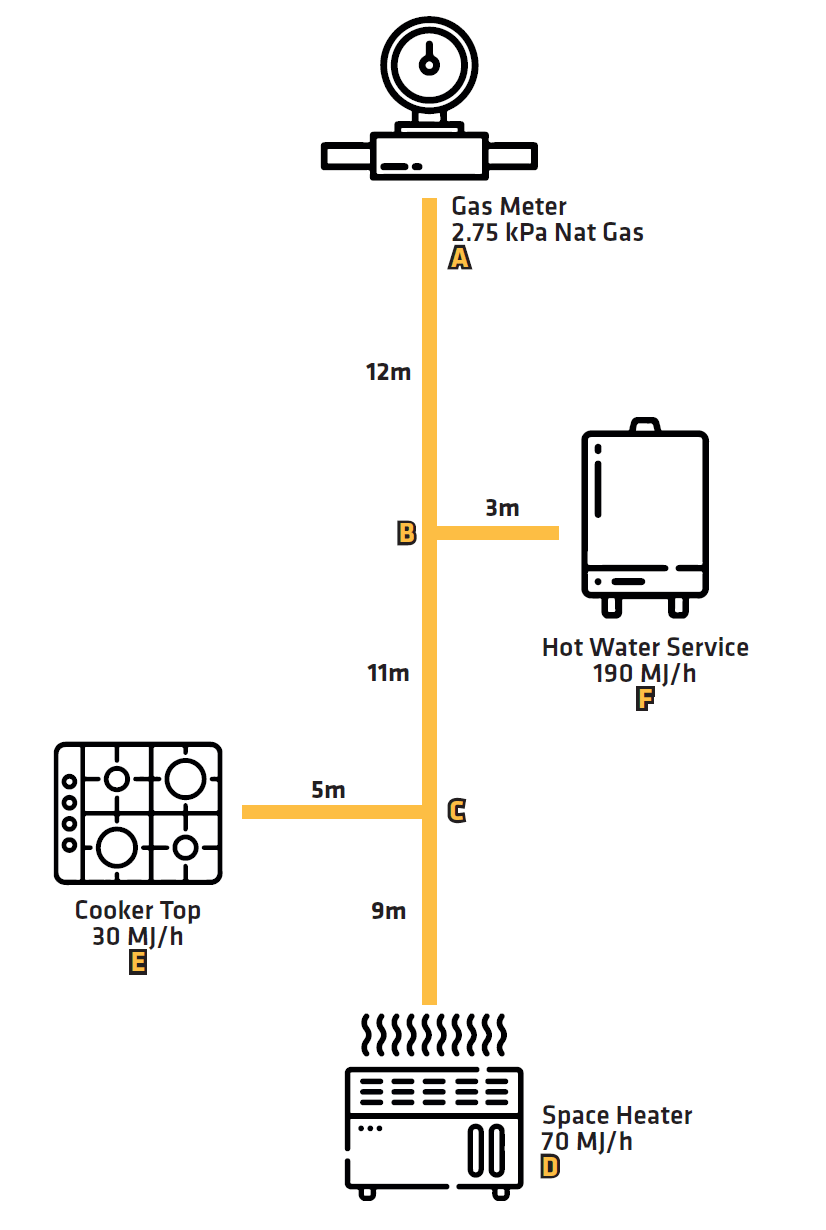# Pipe Sizing Instructions

All Gas installations should be sized correctly to obtain the required flow especially when the system is under full load.

The installations must be installed by a licensed gasfitter and comply to AS/NZS 5601.1:2013.

This example uses Natural Gas with a meter pressure of 2.75kpa and a pressure drop of 0.75kpa.

Step 1 - Draw a schematic of the pipe system and list all the gas data of the appliances (see below diagram).

• Complete gas data in the sizing table (see below small table)

• Label each pipe run section using A-B-C format

• Label the length of each run

• Label the loads of each run

Step 2 - Add the mega joule rating of all the appliances.

Example (190 + 30 + 70) = 290MJ/h

• Refer to the Gasline Sizing table (below large table) to calculate the pipe size of the longest run

• A-B + B-C + C-D (12m + 11m + 9m) = 32m

• Look up the table for the next highest length value (i.e. equal or greater metres value along the top of the table, e.g. 35m)

• Refer to the mega joule rating of all the Appliances (290MJ/h)

• Refer to the Gas Sizing table to work out the pipe size (look down the column to locate the equal or next highest MJ/h rating,

e.g. 438MJ/h, look to the left to the OD column) = 32mm

• Apply this pipe size to run (A-B = 32mm)

Step 3 - Calculate the length of each run:

• For the hot water service (B-F) the calculations are:

A-B + B-F (12m + 3m) = 15m

• Multiply the number of fittings (3) x the fitting equivalent, i.e.: 3 x 0.6 = 1.8

(refer to Gas Sizing Table, large table below), the fittings allowance factor is in the 3rd column of the same row as the pipe size.

• Add the run length to the fitting allowance: 15m + 1.8m = 16.8m

• Look up the table at the next highest length value = 18m

• Look up the mega joule rating of the appliance (190MJ/h)

• Refer to the Gas Sizing table to work out the pipe size = 25mm

• Apply this pipe size to B-F = 25mm

Step 4 - Repeat the above for each of the runs.

For the run B-C, the calculations are: A-B + B-C = (12m + 11m) = 23m

• Multiply the number of fittings (3) x the fitting equivalent (refer to Gas Sizing table, large table below)

• Add the run length to the fitting allowance: 23m + 1.8m = 24.8m

• Look up the table at the next highest length value = 25m

• Add the mega joule rating of the remaining 2 x appliances

(Space heater and Cook top: 70MJ/h + 30MJ/h) = 100MJ/h

• Refer to the Gas Sizing table to work out the pipe size = 20mm

• Apply this pipe size to B-C = 20mm

For the Cooktop run (C-E) the calculations are: A-B + B-C + C-E= (12m + 11m + 5m) = 28m

• Multiply the number of fittings (4) x the fitting equivalent (0.6) correction value (refer to Gas Sizing table, large table below)

• Add the run length to the fitting allowance: 28m + 2.4m = 30.4m

• Look up the table at the next highest length value = 35m

• Refer to mega joule rating of the cooktop appliance (30MJ/h)

• Refer to the Gas Sizing table to work out the pipe size = 16mm

• Apply this pipe size to C-E = 16mm

For the Space Heater run (C-D) the calculations are: A-B + B-C + C-D= (12m + 11m + 9m) = 32m

• Multiply the number of fittings (3) x the fitting equivalent (0.6) correction value (refer to Gas Sizing table, large table below)

• Add the run length to the fitting allowance: 32m + 1.8m = 33.8m

• Look up the table at the next highest length value = 35m

• Refer to mega joule rating of the space heater appliance (70MJ/h)

• Refer to the Gas Sizing table to work out the pipe size = 20mm

• Apply this pipe size to C-D = 20mm

 Pipe Section Gas Flow (MJ/h) Nominal Pipe Size No. of Fittings Used Length of Run (m) A-B 290 32mm N/A 32m B-C 100 20mm 3 24.8m C-D 70 20mm 3 33.8m C-E 30 16mm 4 30.4m B-F 190 25mm 3 16.8m

Gas Pipe Sizing instructions example schematic diagram:### Gas Sizing Table

Please click on the chart below to see the table in full.